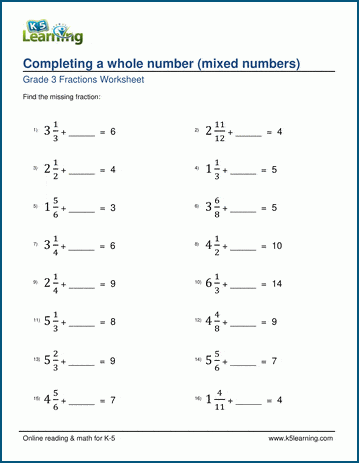# Completing whole numbers

## Fraction worksheets: completing a whole number with mixed numbers

Below are six versions of our grade 3 math worksheet on completing a whole number; students must calculate the number missing from the addition sentence shown.  Answers are shown as mixed numbers.  These worksheets are pdf files.## More fractions worksheets

Explore all of our fractions worksheets, from dividing shapes into "equal parts" to multiplying and dividing improper fractions and mixed numbers.

## What is K5?

K5 Learning offers reading and math worksheets, workbooks and an online reading and math program for kids in kindergarten to grade 5.  We help your children build good study habits and excel in school.